# NCERT Exemplar Solutions: Motion & Time Notes | Study Science Class 7 - Class 7

## Class 7: NCERT Exemplar Solutions: Motion & Time Notes | Study Science Class 7 - Class 7

The document NCERT Exemplar Solutions: Motion & Time Notes | Study Science Class 7 - Class 7 is a part of the Class 7 Course Science Class 7.
All you need of Class 7 at this link: Class 7

Multiple Choice Questions

Q.1. Which of the following cannot be used for measurement of time?
(a) A leaking tap.
(b) Simple pendulum.
(c) Shadow of an object during the day.
Ans:
d
Explanation:
Blinking of the eye is not a periodic phenomenon. Eyes do not blink at a fixed interval of time. Hence Blinking of eyes cannot be used for measurement of time.

Q.2. Two clocks A and B are shown in Figure Clock A has an hour and a minute hand, whereas clock B has an hour hand, minute hand as well as a second hand. Which of the following statement is correct for these clocks?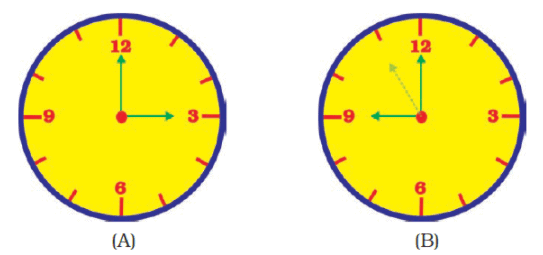(a) A time interval of 30 seconds can be measured by clock A.
(b) A time interval of 30 seconds cannot be measured by clock B.
(c) Time interval of 5 minutes can be measured by both A and B.
(d) Time interval of 4 minutes 10 seconds can be measured by clock A.
Ans:
c
Explanation:
Clock A doesn’t have a seconds hand. Hence seconds cannot be measured by clock A. 5 minutes can be measured by using both the clocks.

Q.3. Two students were asked to plot a distance-time graph for the motion described by Table A and Table B.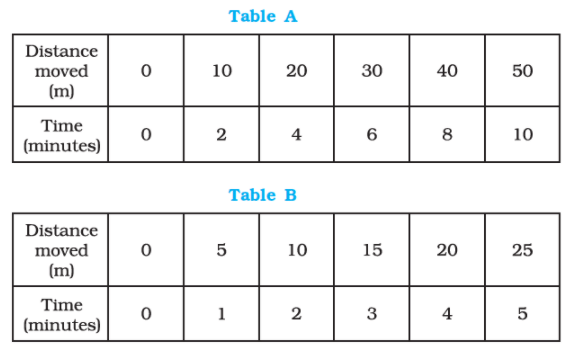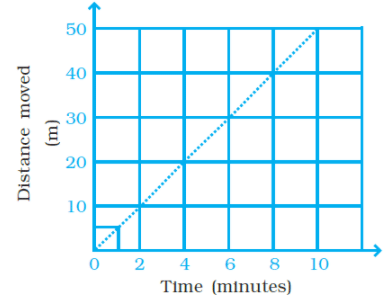The graph given in Figure is true for
(a) both A and B.
(b) A only.
(c) B only.
(d) neither A nor B.
Ans:
a
Explanation:
Since speed for A and B is constant, graph for A and B is a straight line as shown in the figure.

Q.4. A bus travels 54 km in 90 minutes. The speed of the bus is
(a) 0.6 m/s
(b) 10 m/s
(c) 5.4 m/s
(d) 3.6 m/s
Ans:
b
Explanation:
Speed = Distance / Time
Distance = 54km = 54 x1000 = 54000m
Time = 90 minutes = 90×60= 5400s
Speed = 54000/5400 = 10 m/s

Q.5. If we denote speed by S, the distance by D and time by T, the relationship between these quantities is
(a) S = D × T
(b) T = S/D
(c) S = 1/SxD
(c) S = T/D
Ans:
c
Explanation:
Option c) is the correction equation because Speed = Distance/Time

Q.6. Observe the Figure.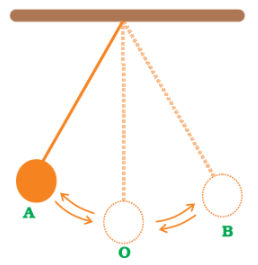The time period of a simple pendulum is the time taken by it to travel from
(a) A to B and back to A.
(b) O to A, A to B and B to A.
(c) B to A, A to B and B to O.
(d) A to B.
Ans:
a

Q.7. Figure shows an oscillating pendulum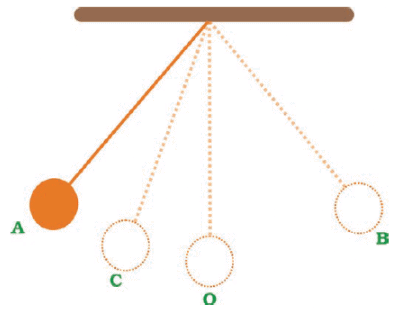Time taken by the bob to move from A to C is t1 and from C to O is t2. The time period of this simple pendulum is
(a) (t1 + t2 )
(b) 2 (t1 + t2 )
(c) 3 (t1 + t2 )
(d) 4 (t1 + t2 )
Ans:
d
Explanation:
The total time taken by the bob to move from A to 0 is (t1+ t2) which is 1/4th time of one full cycle of the pendulum. A time period of pendulum i.e. time taken by pendulum to complete one oscillation from A to B and back to A will be 4 (t1 + t2).

Q.8. The correct symbol to represent the speed of an object is
(a) 5 m/s
(b) 5 mp
(c) 5 m/s-1
(d) 5 s/m
Ans:
a
Explanation:
Unit of speed is meter/ second hence the answer is (a) 5 m/s .

Q.9. Boojho walks to his school which is at a distance of 3 km from his home in 30 minutes. On reaching he finds that the school is closed and comes back by bicycle with his friend and reaches home in 20 minutes. His average speed in km/h is
(a) 8.3
(b) 7.2
(c) 5
(d) 3.6
Ans: b
Explanation: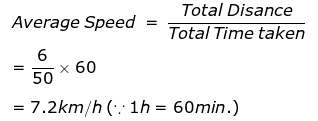Q.1. A simple pendulum is oscillating between two points A and B as shown in Figure. Is the motion of the bob uniform or non-uniform?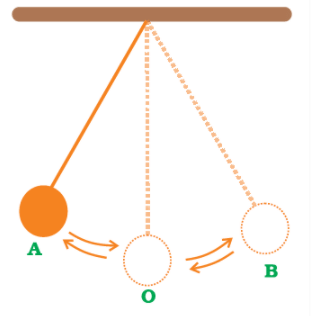Ans: The motion of the bob is non-uniform because the speed of the bob keeps changing.

Q.2. Paheli and Boojho have to cover different distances to reach their school but they take the same time to reach the school. What can you say about their speed?
Ans: Because their speed will be different from one another.

Q.3. If Boojho covers a certain distance in one hour and Paheli covers the same distance in two hours, who travels in a higher speed?

Ans: Boojho travels in a higher speed, hence he reaches the distance early.

Q.1. Complete the data of the table given below with the help of the distance-time graph given in Figure.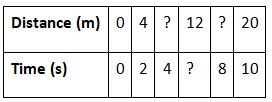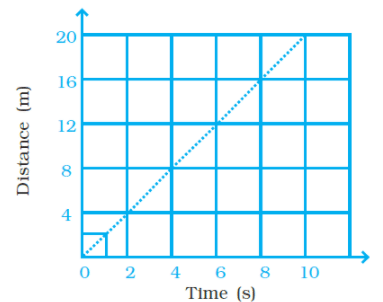Ans: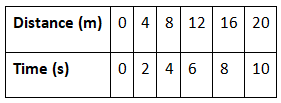Q.2. The average age of children in Class VII is 12 years and 3 months. Express this age in seconds.
Ans:
12 years 3 months
= 12 × 365 + 3 × 30 = 4470 days
= 4470 × 24 × 60 × 60 s = 386208000 s

Q.3. A spaceship travels 36,000 km in one hour. Express its speed in km/s.
Ans: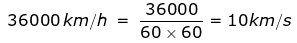Q.4. Starting from A, Paheli moves along a rectangular path ABCD as shown in Figure. She takes 2 minutes to travel each side. Plot a distance-time graph and explain whether the motion is uniform or non-uniform.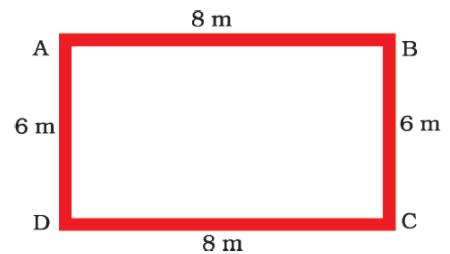Ans:
Since the distance covered per unit time for the entire distance covered is not the same, the motion is non-uniform.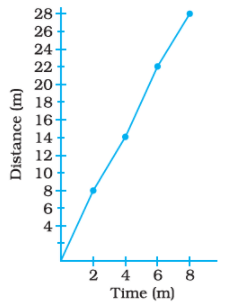Q.5. Plot a distance-time graph of the tip of the second hand of a clock by selecting 4 points on x-axis and y-axis respectively. The circumference of the circle traced by the second hand is 64 cm.
Ans: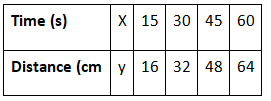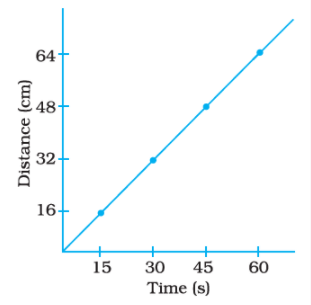Q.1. Given below as Figure is the distance-time graph of the motion an object.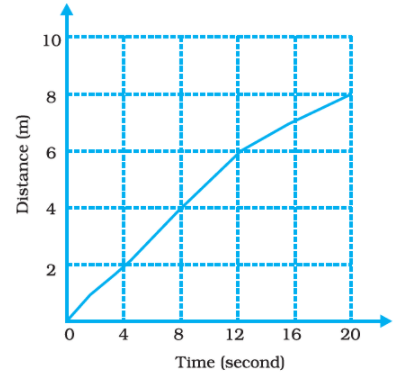(i) What will be the position of the object at the 20s?
(ii) What will be the distance travelled by the object in 12s?
(iii) What is the average speed of the object?

Ans:

1. At 20s, the object will be 8 m away from the starting point.
2. In 12s, distance travelled by the object will be 6 m.
3. Average speed of the object Total distance=Total distance/Time taken=8m/20s=0.4m/s

Q.2. Distance between Bholu’s and Golu’s house is 9 km. Bholu has to attend Golu’s birthday party at 7 o’clock. He started from his home at 6 o’clock on his bicycle and covered a distance of 6 km in 40 minutes. At that point, he met Chintu and he spoke to him for 5 minutes and reached Golu’s birthday party at 7 o’clock. With what speed did he cover the second part of the journey? Calculate his average speed for the entire journey.

The speed with which Bholu covered the second part of the journey
Ans: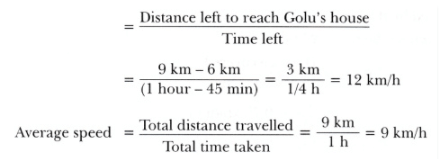Q.3. Boojho goes to the football ground to play football. The distance-time graph of his journey from his home to the ground is given as Figure.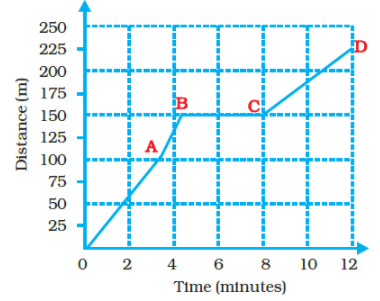(a) What does the graph between point B and C indicate about the motion of Boojho?
(b) Is the motion between 0 to 4 minutes uniform or nonuniform?
(c) What is his speed between 8 and 12 minutes of his journey?
Ans:
(a) Boojho’s speed is zero hence he will be in rest
(b) motion between 0 to 4 minutes will be nonuniform
(c) 75/4 = 18.75 m/minute

The document NCERT Exemplar Solutions: Motion & Time Notes | Study Science Class 7 - Class 7 is a part of the Class 7 Course Science Class 7.
All you need of Class 7 at this link: Class 7Use Code STAYHOME200 and get INR 200 additional OFF

## Science Class 7

161 videos|216 docs|54 tests

Track your progress, build streaks, highlight & save important lessons and more!

,

,

,

,

,

,

,

,

,

,

,

,

,

,

,

,

,

,

,

,

,

;